Question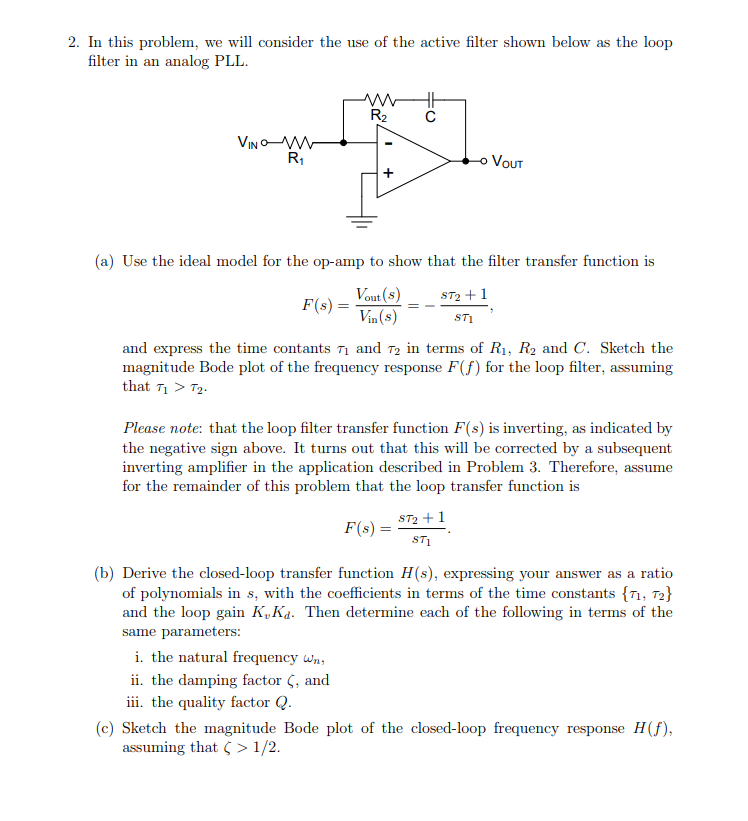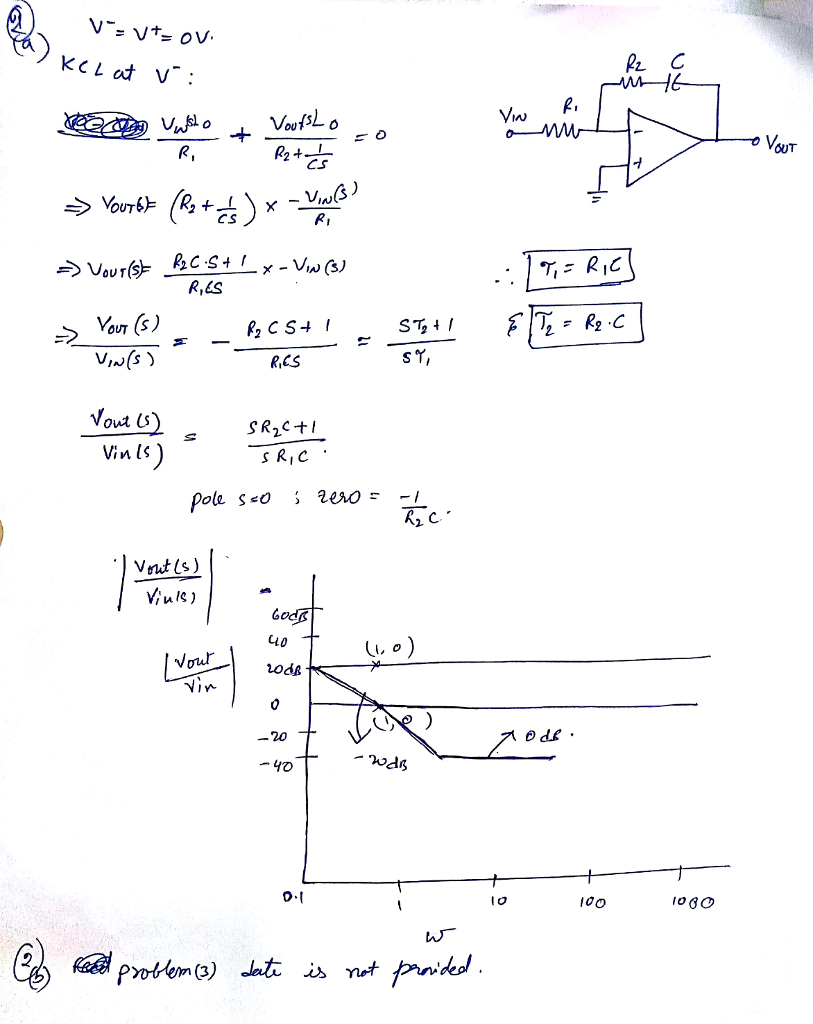#### Earn Coins

Coins can be redeemed for fabulous gifts.

Similar Homework Help Questions
• ### Problem 4. Consider the control system shown below with plant G(s) that has time con- stants...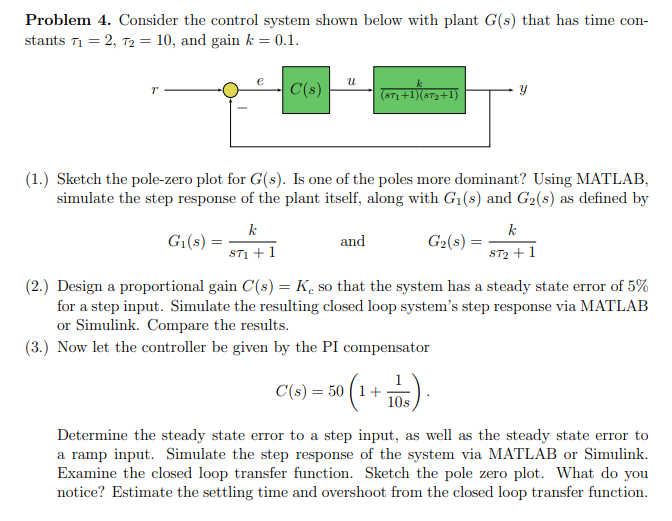Problem 4. Consider the control system shown below with plant G(s) that has time con- stants T1 = 2, T2 = 10, and gain k = 0.1. 4 673 +1679+1) (1.) Sketch the pole-zero plot for G(s). Is one of the poles more dominant? Using MATLAB, simulate the step response of the plant itself, along with G1(s) and G2(s) as defined by Gl(s) = and G2(s) = sti + 1 ST2+1 (2.) Design a proportional gain C(s) = K so...

• ### Consider the system shown as below. Draw a Bode diagram of the open-loop transfer function G(s).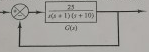1 Consider the system shown as below. Draw a Bode diagram of the open-loop transfer function G(s). Determine the phase margin, gain-crossover frequency, gain margin and phase-crossover frequency, (Sketch the bode diagram by hand) 2 Consider the system shown as below. Use MATLAB to draw a bode diagram of the open-loop transfer function G(s). Show the gain-crossover frequency and phase-crossover frequency in the Bode diagram and determine the phase margin and gain margin. 3. Consider the system shown as below. Design a...

• ### Problem 3 A boat of mass m glides through the water, experiencing viscous fluid resistance with...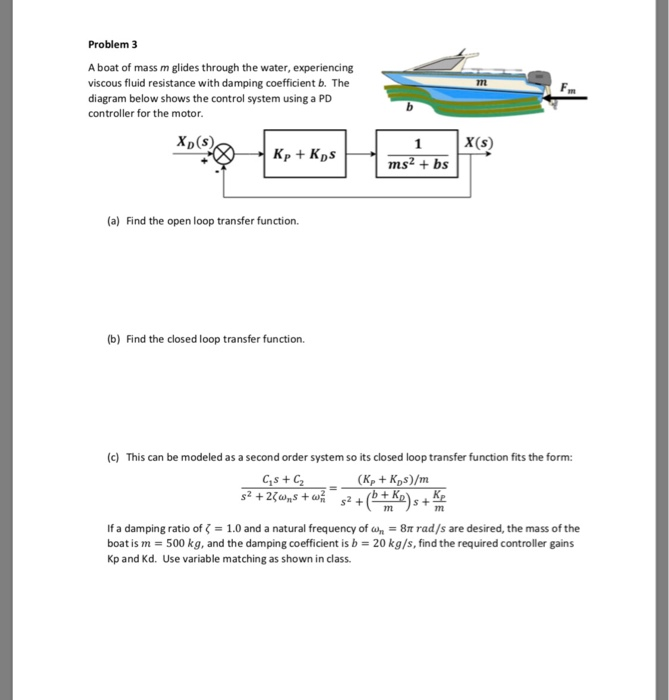Problem 3 A boat of mass m glides through the water, experiencing viscous fluid resistance with damping coefficient b. The diagram below shows the control system using a PD controller for the motor. 1n XD (s) 1 X(s) (a) Find the open loop transfer function. (b) Find the closed loop transfer function. (c) This can be modeled as a second order system so its closed loop transfer function fits the form: Cs C2 If a dam ping ratio of ζ...

• ### Consider the following transfer function of a bandpass filter: 20 1,500 T(S) = 2 1,500 +...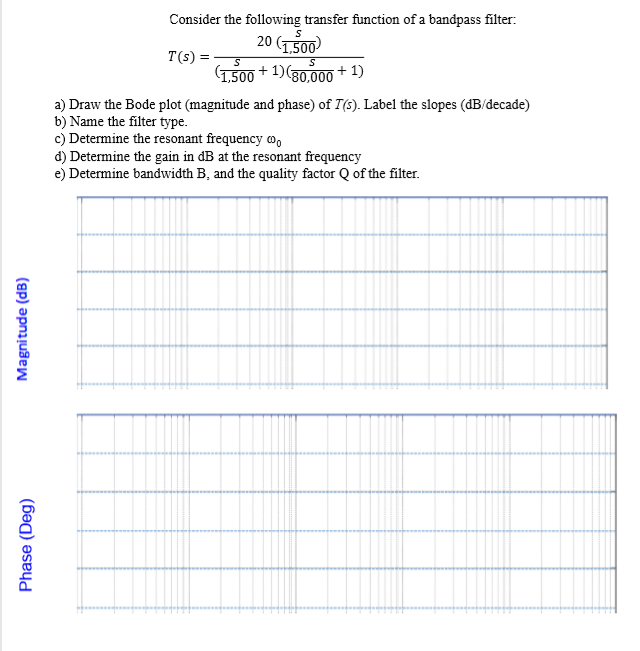Consider the following transfer function of a bandpass filter: 20 1,500 T(S) = 2 1,500 + 1)(30.000 +1) a) Draw the Bode plot (magnitude and phase) of T(s). Label the slopes (dB/decade) b) Name the filter type. c) Determine the resonant frequency o d) Determine the gain in dB at the resonant frequency e) Determine bandwidth B, and the quality factor of the filter. Magnitude (dB) Phase (Deg)

• ### 3. For the active filter circuit below, complete the following: a) Find the magnitude of the...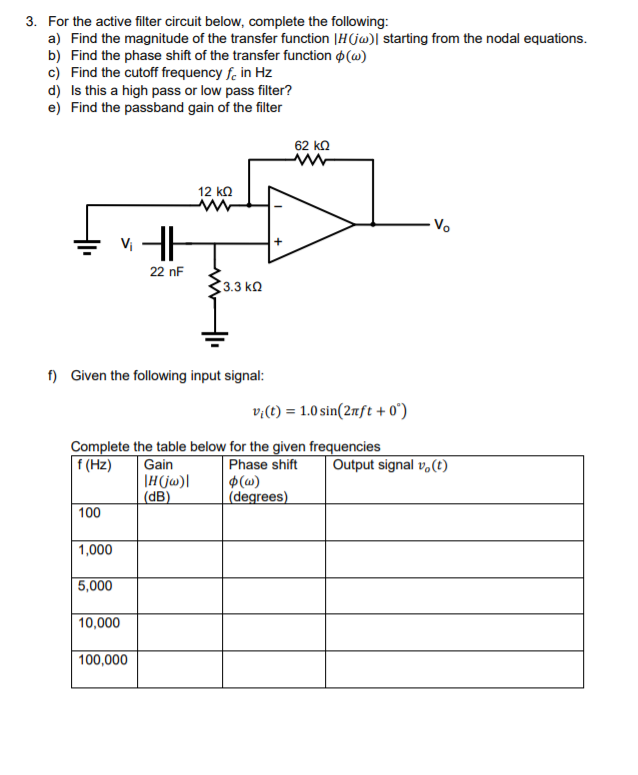3. For the active filter circuit below, complete the following: a) Find the magnitude of the transfer function | H | starting from the nodal equations. b) Find the phase shift of the transfer function (W) c) Find the cutoff frequency fc in Hz d) Is this a high pass or low pass filter? e) Find the passband gain of the filter 62 k2 ANA EVA 22 nF 3.3k f) Given the following input signal: vi(t) = 1.0 sin(2nft +...

• ### Consider the unity-feedback system shown below: R(s) E(s) input: r(t), output: y(t) C(s) P(s) error: e()...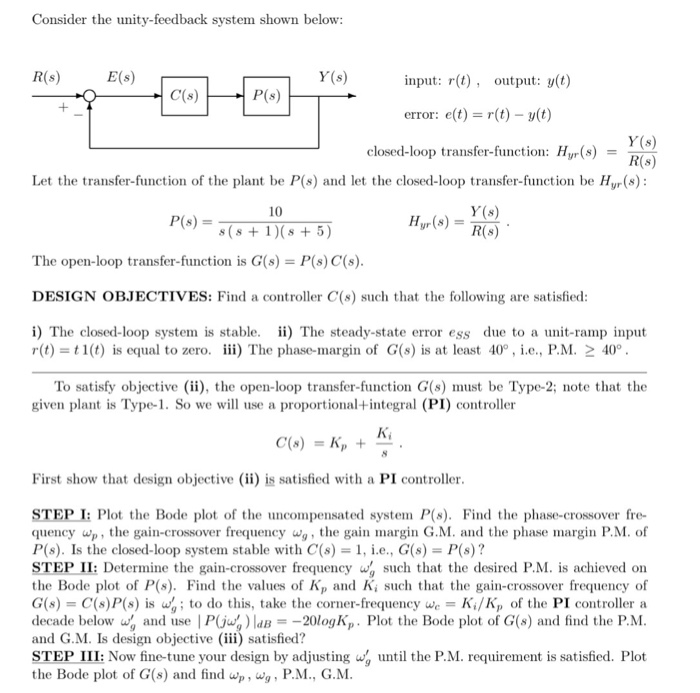Consider the unity-feedback system shown below: R(s) E(s) input: r(t), output: y(t) C(s) P(s) error: e() r(t) y(t) closed-loop transfer-function: Hyr(sD t the closed-loop transfer-function be Hyr(s) Y (s) R(s) Let the transfer-function of the plant be P(s) 10 s (s 1) (s 5) The open-loop transfer-function is G(s) P(s) C(s) DESIGN OBJECTIVES: Find a controller C(s) such that the following are satisfied i) The closed-loop system is stable. ii) The steady-state error ess due to a unit-ramp input r(t)...

• ### Consider the filter circuit (Figure 1) with R=500 N and C ==uF. Learning Goal: To understand...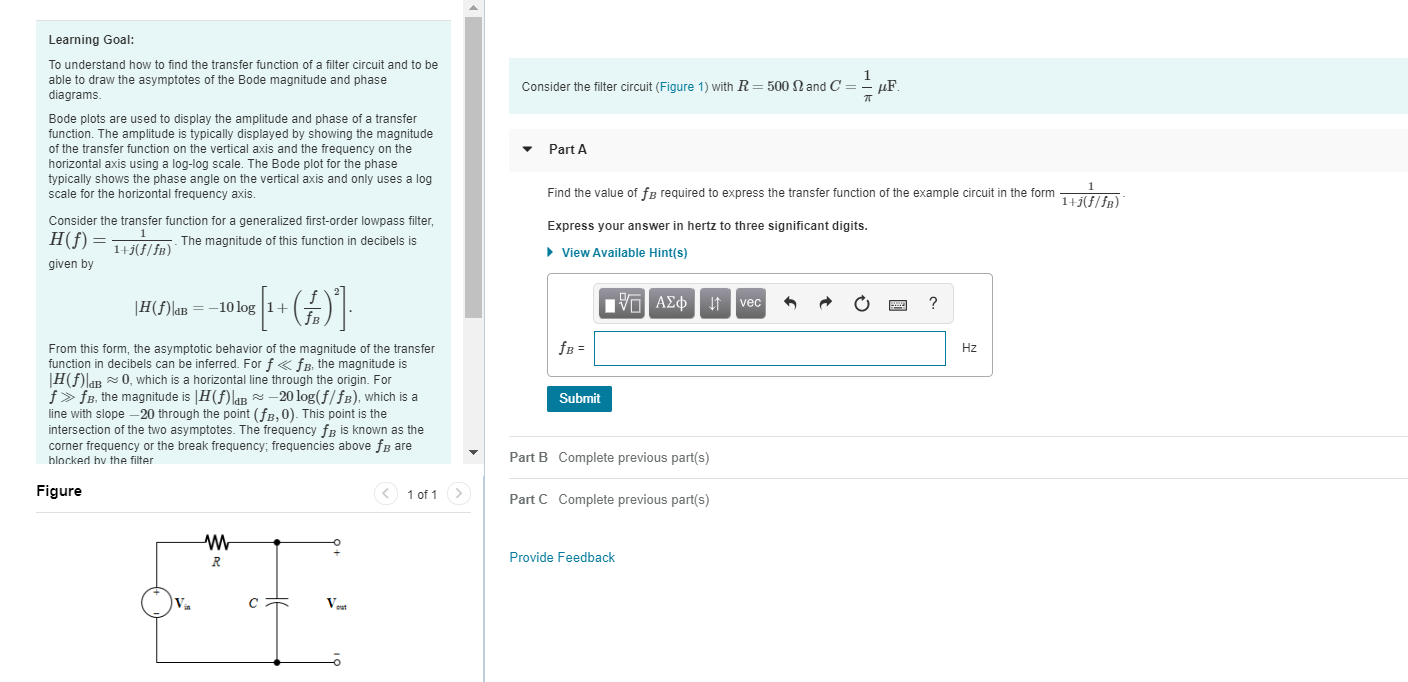Consider the filter circuit (Figure 1) with R=500 N and C ==uF. Learning Goal: To understand how to find the transfer function of a filter circuit and to be able to draw the asymptotes of the Bode magnitude and phase diagrams Bode plots are used to display the amplitude and phase of a transfer function. The amplitude is typically displayed by showing the magnitude of the transfer function on the vertical axis and the frequency on the horizontal axis using...

• ### Problem 2 a) Using 5 nF capacitors, design an active broad- band first-order bandreject filter with...

Problem 2 a) Using 5 nF capacitors, design an active broad- band first-order bandreject filter with a lower cutoff frequency of 1000 Hz, an upper cut-off frequency of 5000 Hz, and a pass band gain of 10dB. b) Draw the schematic diagram of the filter. c) Write the transfer function to find H(jωo), where ωo is the center frequency of the filter. d) What is the gain (in decibels) of the filer at the center frequency? e) Using Matlab make...

• ### The center frequency is not given. I believe that it must be find based on the body plot. Problem 6:The Bode plot for a passive series RLC bandpass filter is shown in Fig. 2. This filter was built...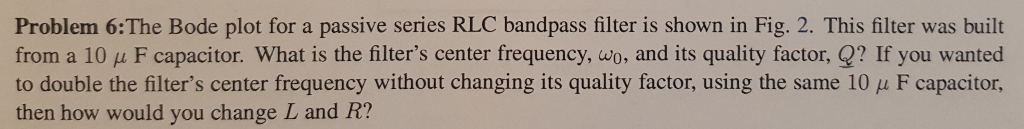The center frequency is not given. I believe that it must be find based on the body plot. Problem 6:The Bode plot for a passive series RLC bandpass filter is shown in Fig. 2. This filter was built from a 10 μ F capacitor. What is the filter's center frequency, wo, and its quality factor,昱? If you wanted to double the filter's center frequency without changing its quality factor, using the same 10 pu F capacitor, then how would you...

• ### Please answer all parts thank you 6. [15 POINTS] Consider a passive low-pass filter as shown...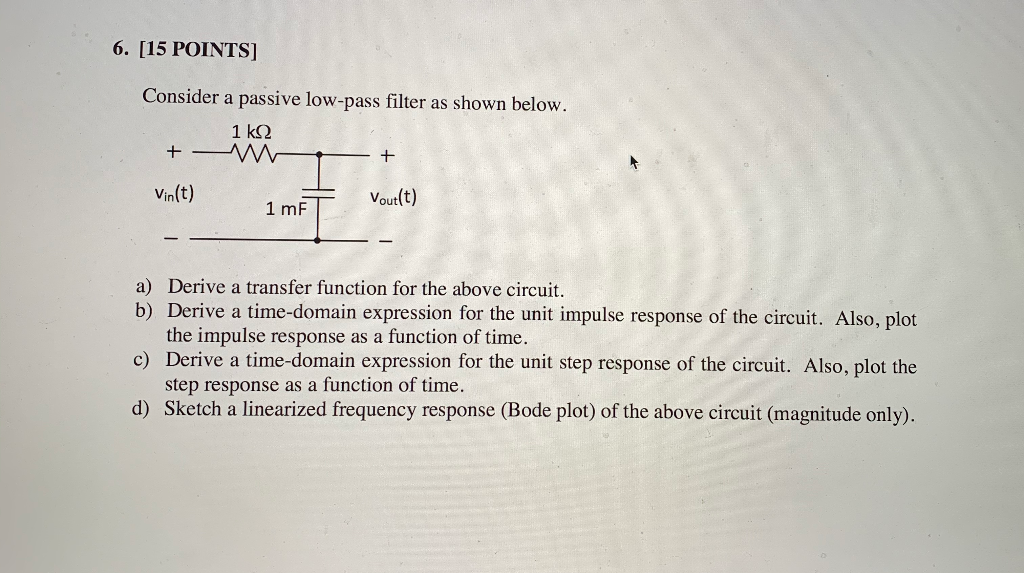Please answer all parts thank you 6. [15 POINTS] Consider a passive low-pass filter as shown below. 1k2 + - m - + Vin(t) 1 mF Vout(t) a) Derive a transfer function for the above circuit. b) Derive a time-domain expression for the unit impulse response of the circuit. Also, plot the impulse response as a function of time. c) Derive a time-domain expression for the unit step response of the circuit. Also, plot the step response as a function...